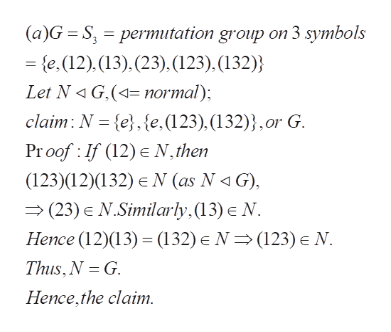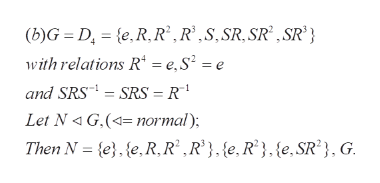# Find all normal subgroups of G where(a) G = S3,(b) G = D4, the group of symmetries of the square,(c) G = Q4, the quaternion group

Question
1 views

Find all normal subgroups of G where
(a) G = S3,

(b) G = D4, the group of symmetries of the square,

(c) G = Q4, the quaternion group

check_circle

Step 1

To find all the normal subgroups of the given (three) groups

Step 2

(a) the only normal subgroups are the triivial, the alternating and the whole grouphelp_outlineImage Transcriptionclose(a)G S, permutation group on 3 symbols - e.(12), (13). (23), (123). (132) Let N 4 G,(normal) claim N e, {e,(123).(132)},or G Proof If (12) N, then (123)(12)(132) N (as N G) (23) E N.Similarly.(13) E N Hence (12)(13) (132) e N (123) N Thus,N G Hence,the claim. fullscreen
Step 3

There are five normal subgroups of G. The obvious ones are the identity, the subgroup generated by the rotation R , (as it is a subgroup of order 2 in G), and t...help_outlineImage Transcriptionclose(b)G D, {e.R.R, R',S,SR. SR* ,SR} with relations R = e,S2 = e and SRS SRS = R Let N G,(normal) Then N e e,R, R2 R}, {e, R},(e,SR'}, G. fullscreen

### Want to see the full answer?

See Solution

#### Want to see this answer and more?

Solutions are written by subject experts who are available 24/7. Questions are typically answered within 1 hour.*

See Solution
*Response times may vary by subject and question.
Tagged in

### Math